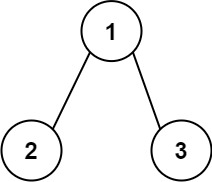# 129. Sum Root to Leaf Numbers

Medium
You are given the `root` of a binary tree containing digits from `0` to `9` only.
Each root-to-leaf path in the tree represents a number.
• For example, the root-to-leaf path `1 -> 2 -> 3` represents the number `123`.
Return the total sum of all root-to-leaf numbers. Test cases are generated so that the answer will fit in a 32-bit integer.
A leaf node is a node with no children.
Example 1:Input: root = [1,2,3]
Output:
25
Explanation:
The root-to-leaf path 1->2 represents the number 12.
The root-to-leaf path 1->3 represents the number 13.
Therefore, sum = 12 + 13 = 25.
Example 2:Input: root = [4,9,0,5,1]
Output:
1026
Explanation:
The root-to-leaf path 4->9->5 represents the number 495.
The root-to-leaf path 4->9->1 represents the number 491.
The root-to-leaf path 4->0 represents the number 40.
Therefore, sum = 495 + 491 + 40 = 1026.
Constraints:
• The number of nodes in the tree is in the range `[1, 1000]`.
• `0 <= Node.val <= 9`
• The depth of the tree will not exceed `10`.

### 解題

Runtime: 0 ms, faster than 100%
Memory Usage: 2.2 MB, less than 77.70%
/**
* Definition for a binary tree node.
* type TreeNode struct {
* Val int
* Left *TreeNode
* Right *TreeNode
* }
*/
func sumNumbers(root *TreeNode) int {
return helper(root, 0)
}
func helper(root *TreeNode, sum int) int {
if root == nil { return 0 }
if root.Right == nil && root.Left == nil {
return sum*10 + root.Val
}
lsum := helper(root.Left, sum*10 + root.Val)
rsum := helper(root.Right, sum*10 + root.Val)
return lsum + rsum
}